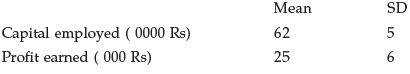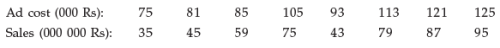Courses

# Test: Correlation And Regression- 4

## 40 Questions MCQ Test Quantitative Aptitude for CA CPT | Test: Correlation And Regression- 4

Description
This mock test of Test: Correlation And Regression- 4 for CA Foundation helps you for every CA Foundation entrance exam. This contains 40 Multiple Choice Questions for CA Foundation Test: Correlation And Regression- 4 (mcq) to study with solutions a complete question bank. The solved questions answers in this Test: Correlation And Regression- 4 quiz give you a good mix of easy questions and tough questions. CA Foundation students definitely take this Test: Correlation And Regression- 4 exercise for a better result in the exam. You can find other Test: Correlation And Regression- 4 extra questions, long questions & short questions for CA Foundation on EduRev as well by searching above.
QUESTION: 1

Solution:
QUESTION: 2

Solution:
QUESTION: 3

### The two lines of regression are given by 8x + 10y = 25 and 16x + 5y = 12 respectively.   Q. If the variance of x is 25, what is the standard deviation of y?

Solution:
QUESTION: 4

Given below the information about the capital employed and profit earned by a company over the last twenty five years:Q. Correlation Coefficient between capital and profit = 0.92. The sum of the Regression coefficients for the above data would be:

Solution:
QUESTION: 5

The coefficient of correlation between cost of advertisement and sales of a product on the basis of the following data:Solution:
QUESTION: 6

–—————— is concerned with the measurement of the “strength of association” between variables.

Solution:
QUESTION: 7

—————— gives the mathematical relationship of the variables.

Solution:
QUESTION: 8

When high values of one variable are associated with high values of the other & low values of one variable are associated with low values of another, then they are said to be

Solution:
QUESTION: 9

If high values of one tend to low values of the other, they are said to be

Solution:
QUESTION: 10

Correlation coefficient between two variables is a measure of their linear relationship .

Solution:
QUESTION: 11

Correlation coefficient is dependent of the choice of both origin & the scale of observations.

Solution:
QUESTION: 12

Correlation coefficient is a pure number.

Solution:
QUESTION: 13

Correlation coefficient is —————— of the units of measurement.

Solution:
QUESTION: 14

The value of correlation coefficient lies between

Solution:
QUESTION: 15

Correlation coefficient can be found out by

Solution:
QUESTION: 16

Covariance measures _________ variations of two variables.

Solution:
QUESTION: 17

In calculating the Karl Pearson’s coefficient of correlation it is necessary that the data should be of numerical measurements. The statement is

Solution:
QUESTION: 18

Rank correlation coefficient lies between

Solution:
QUESTION: 19

A coefficient near +1 indicates tendency for the larger values of one variable to be associated with the larger values of the other.

Solution:
QUESTION: 20

In rank correlation coefficient the association need not be linear.

Solution:
QUESTION: 21

In rank correlation coefficient only an increasing/decreasing relationship is required.

Solution:
QUESTION: 22

Great advantage of ____________ is that it can be used to rank attributes which can not be expressed by way of numerical value.

Solution:
QUESTION: 23

The sum of the difference of rank is

Solution:
QUESTION: 24

Karl Pearson’s coefficient is defined from

Solution:
QUESTION: 25

Correlation methods are used to study the relationship between two time series of data which are recorded annually, monthly, weekly, daily and so on.

Solution:
QUESTION: 26

Age of Applicants for life insurance and the premium of insurance – correlations are

Solution:
QUESTION: 27

“Unemployment index and the purchasing power of the common man“ ——Correlations are

Solution:
QUESTION: 28

Production of pig iron and soot content in Durgapur – Correlations are

Solution:
QUESTION: 29

“Demand for goods and their prices under normal times” —— Correlations are

Solution:
QUESTION: 30

___________ is a relative measure of association between two or more variables.

Solution:
QUESTION: 31

The line of regression passes through the points, bearing _________ no. of points on both sides

Solution:
QUESTION: 32

Under Algebraic Method we get ————— linear equations.

Solution:
QUESTION: 33

In linear equations Y = a + bX and X= a + bY ‘a‘ is the

Solution:
QUESTION: 34

In linear equations Y = a + bX and X = a + bY ‘ b ‘ is the

Solution:
QUESTION: 35

The equations Y = a + bX and X = a + bY are based on the method of

Solution:
QUESTION: 36

The line Y = a + bX represents the regression equation of

Solution:
QUESTION: 37

The line X = a + bY represents the regression equation of

Solution:
QUESTION: 38

Two regression lines always intersect at the means.

Solution:
QUESTION: 39

r, bxy , byx all have ______ sign.

Solution:
QUESTION: 40

The regression coefficients are zero if r is equal to

Solution: